# How to Calculate and Solve for Crude Oil Gravity, Oil Density and Water Density | The Calculator Encyclopedia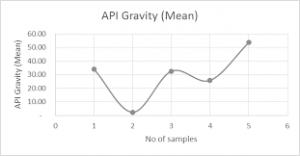The image above represents crude oil gravity.

To compute for the crude oil gravity, two essential parameters are needed and these parameters are oil density (ρo) and water density (ρw).

The formula for calculating the crude oil gravity:

γo = ρo / ρw

Where;

γo = Crude Oil Gravity
ρo = Oil Density
ρw = Water Density

Let’s solve an example;
Find the crude oil gravity when the oil density is 28 and the water density is 46.

This implies that;

ρo = Oil Density = 28
ρw = Water Density = 46

γo = ρo / ρw
γo = 28 / 46
γo = 0.608

Therefore, the crude oil density is 0.608.

Calculating the Oil Density when the Crude Oil Gravity and the Water Density is Given.

ρo = γo x ρw

Where;

ρo = Oil Density
γo = Crude Oil Gravity
ρw = Water Density

Let’s solve an example;
Find the oil density when the crude oil gravity is 30 and the water density is 16.

This implies that;

γo = Crude Oil Gravity = 30
ρw = Water Density = 16

ρo = γo x ρw
ρo = 30 x 16
ρo = 480

Therefore, the oil density is 480.

Calculating the Water Density when the Crude Oil Density and the Oil Density is Given.

ρw = ρo / γo

Where;

ρw = Water Density
γo = Crude Oil Gravity
ρo = Oil Density

Let’s solve an example;
Find the water density when the oil density is 32 and the crude oil gravity is 12.

This implies that;

γo = Crude Oil Gravity = 12
ρo = Oil Density = 32

ρw = ρo / γo
ρw = 32 / 12
ρw = 2.667

Therefore, the water density is 2.667.

Nickzom Calculator – The Calculator Encyclopedia is capable of calculating the crude oil gravity.

To get the answer and workings of the crude oil gravity using the Nickzom Calculator – The Calculator Encyclopedia. First, you need to obtain the app.

You can get this app via any of these means:

To get access to the professional version via web, you need to register and subscribe for NGN 1,500 per annum to have utter access to all functionalities.
You can also try the demo version via https://www.nickzom.org/calculator

Android (Paid) – https://play.google.com/store/apps/details?id=org.nickzom.nickzomcalculator
Android (Free) – https://play.google.com/store/apps/details?id=com.nickzom.nickzomcalculator
Apple (Paid) – https://itunes.apple.com/us/app/nickzom-calculator/id1331162702?mt=8
Once, you have obtained the calculator encyclopedia app, proceed to the Calculator Map, then click on Petroleum under EngineeringNow, Click on Fluid Properties under Petroleum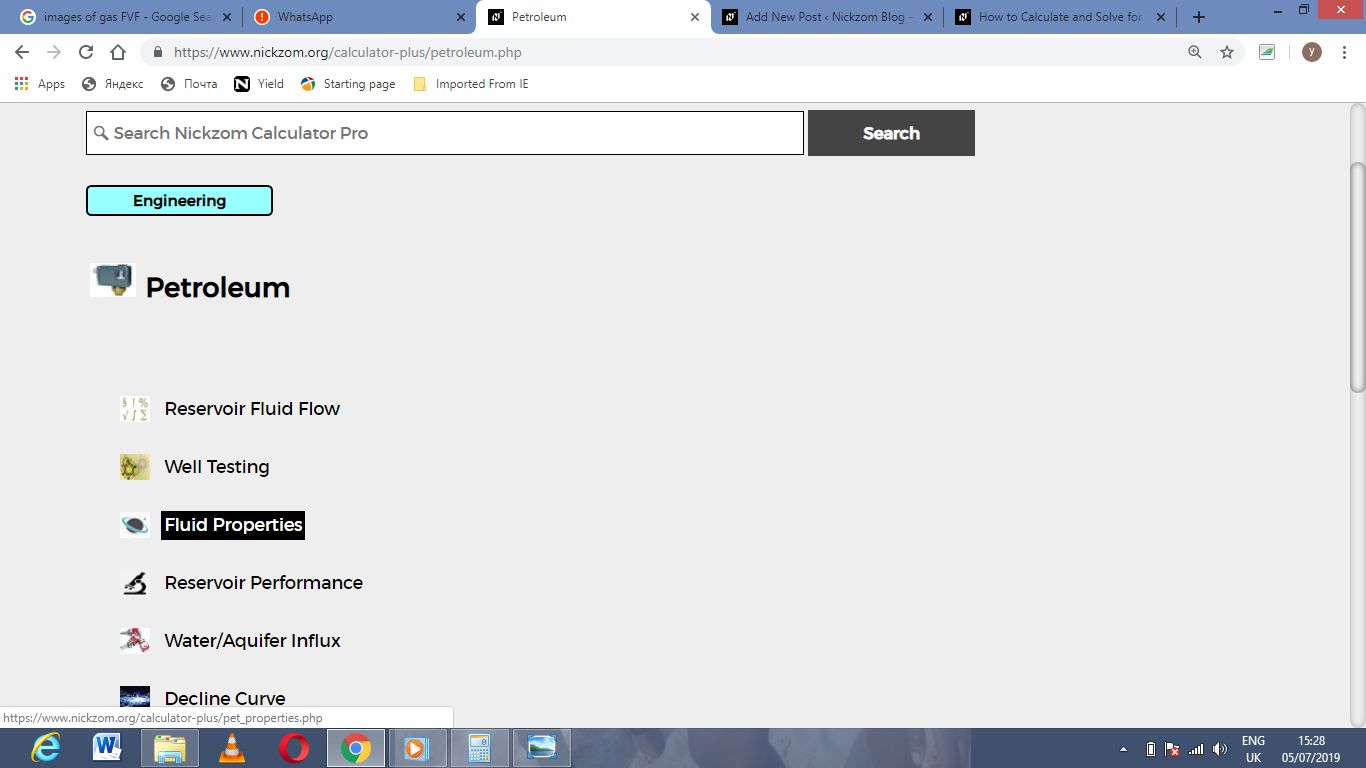Now, Click on Crude Oil Gravity under Fluid Properties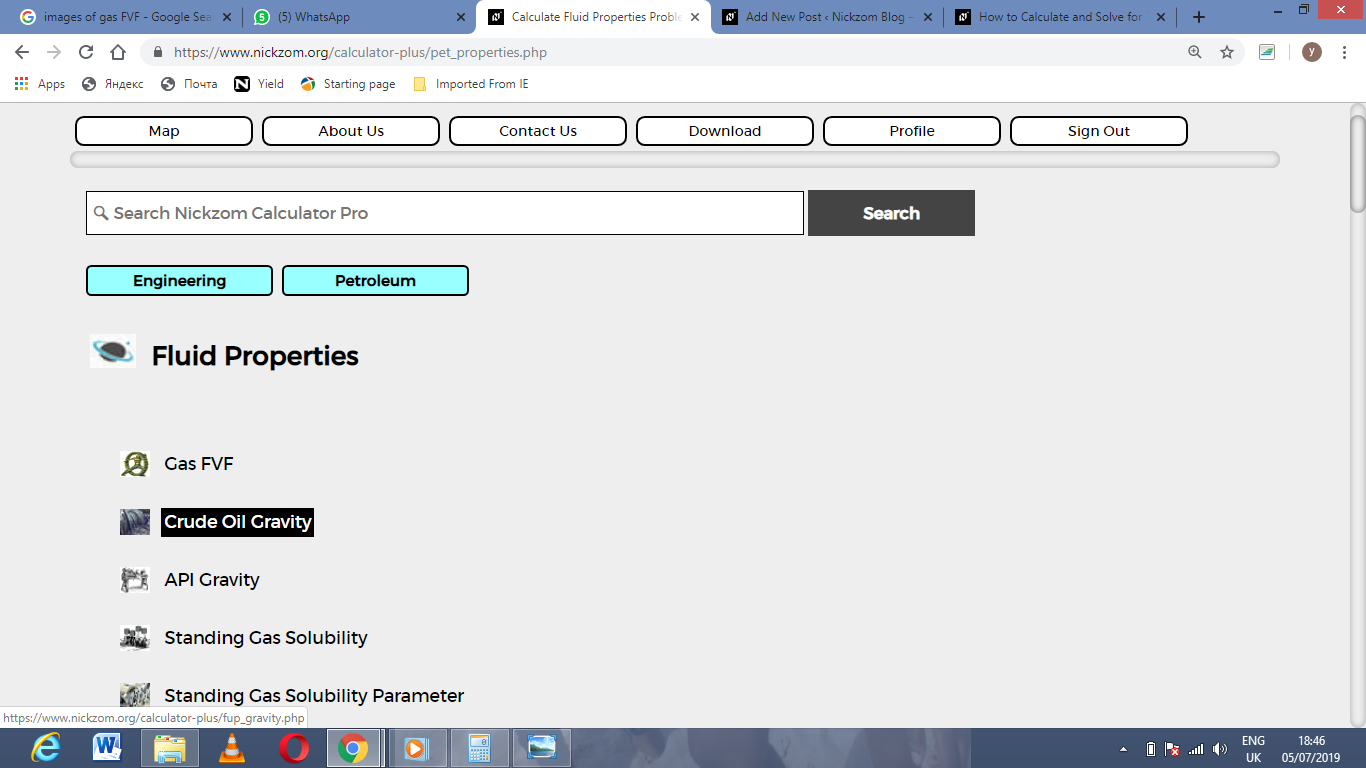The screenshot below displays the page or activity to enter your values, to get the answer for the crude oil gravity according to the respective parameters which are the oil density (ρo) and water density (ρw).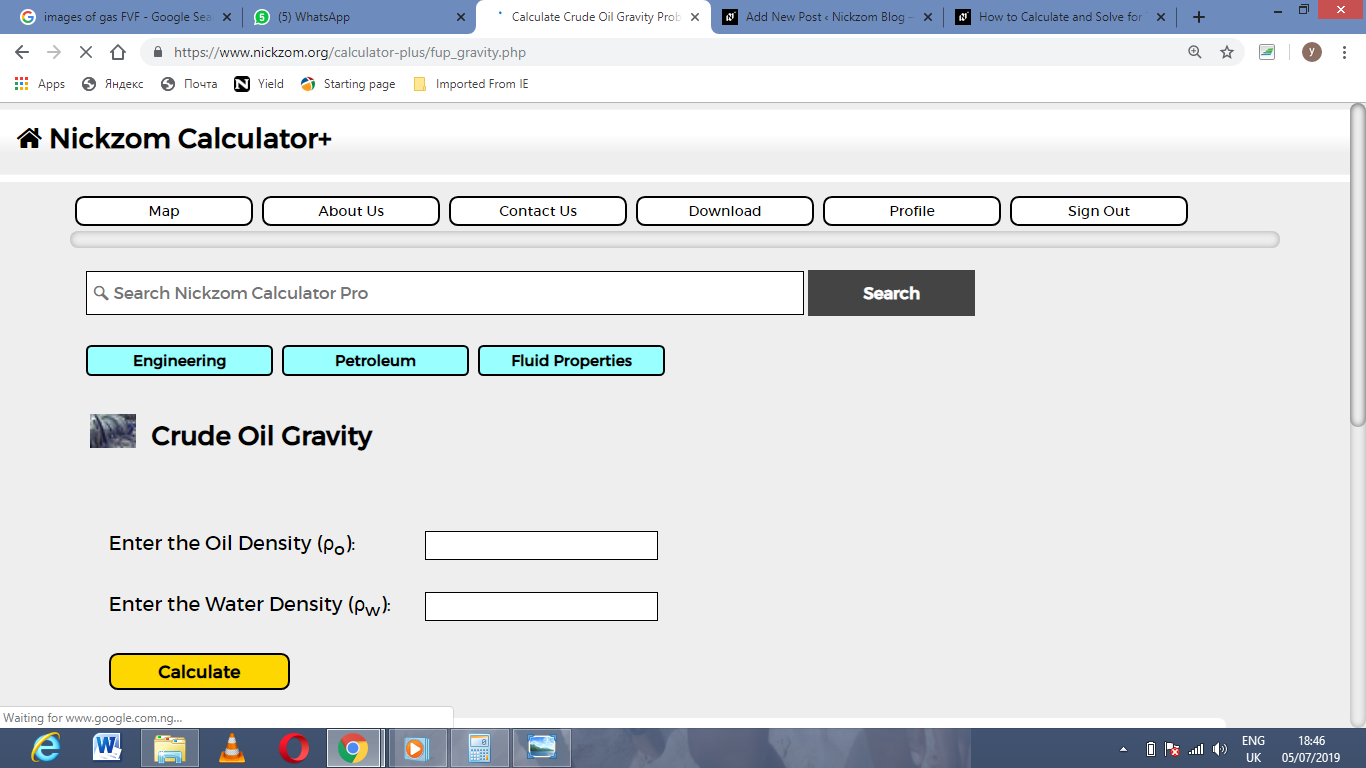Now, enter the values appropriately and accordingly for the parameters as required by the oil density (ρo) is 28 and water density (ρw) is 46.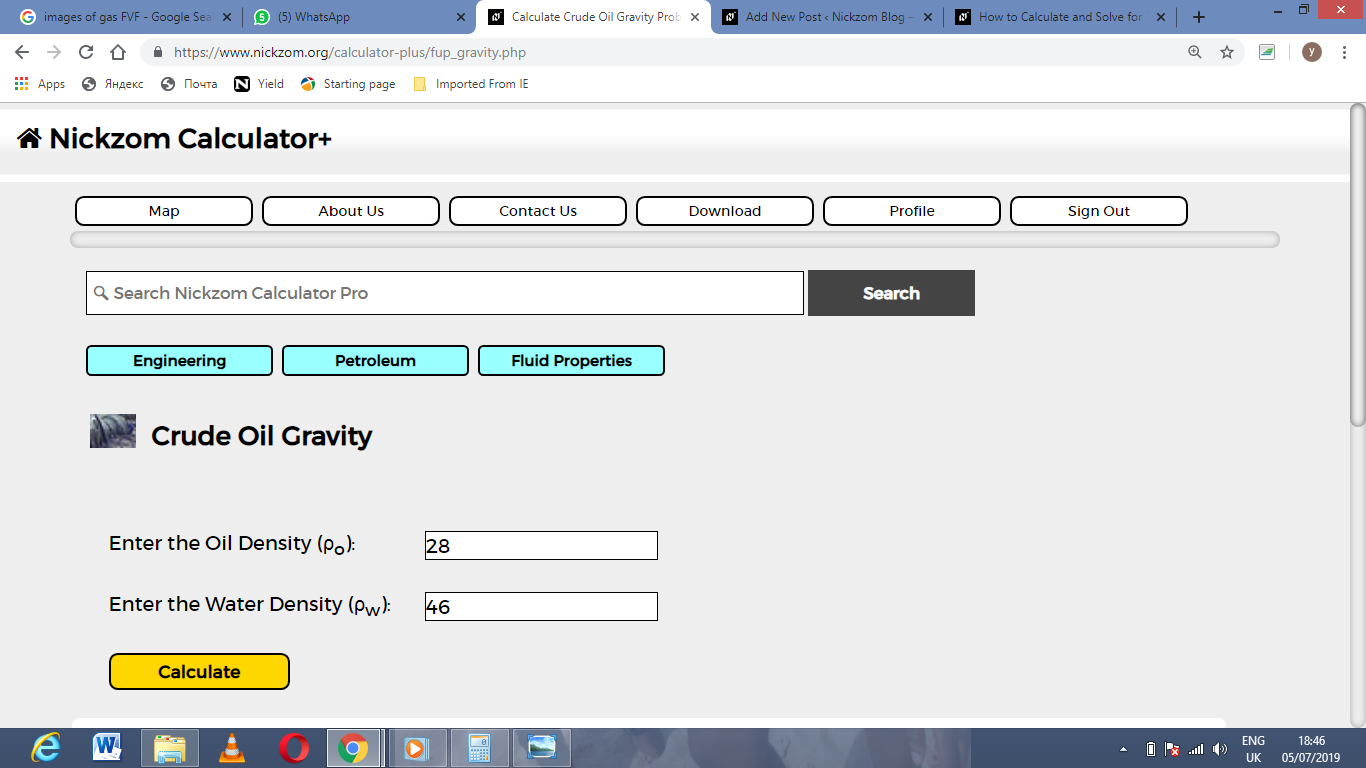Finally, Click on Calculate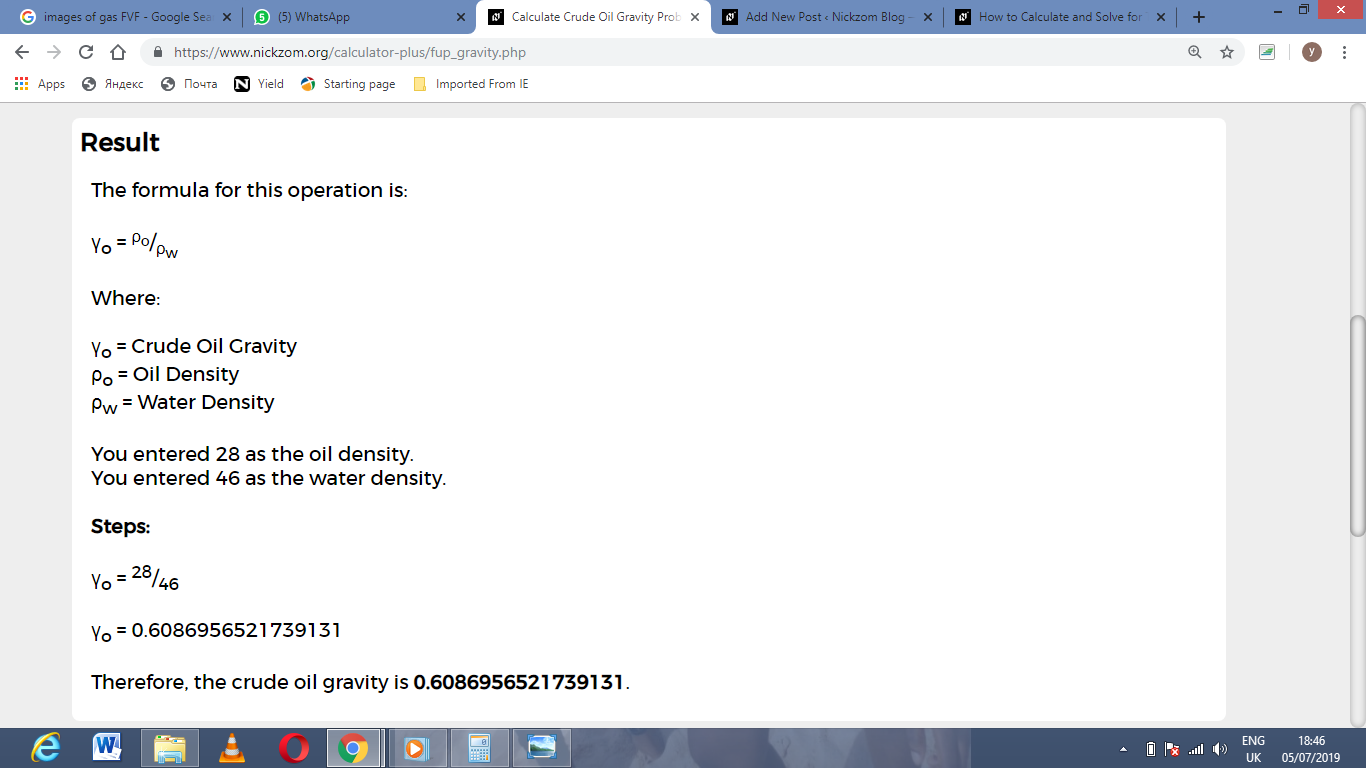As you can see from the screenshot above, Nickzom Calculator– The Calculator Encyclopedia solves for the crude oil gravity and presents the formula, workings and steps too.